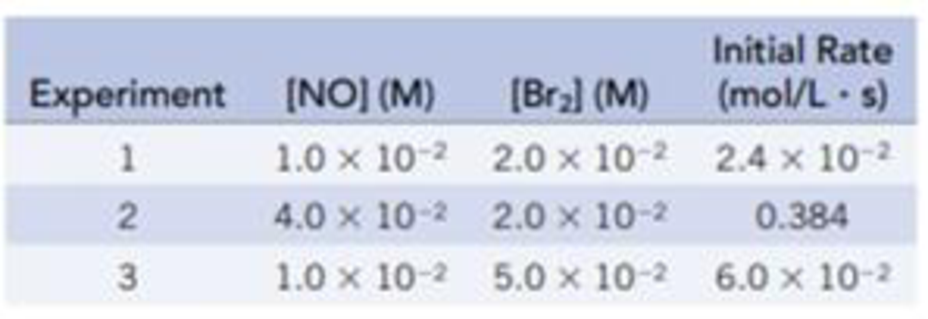Chapter 14, Problem 56GQ

Chapter
Section
Textbook Problem

Data for the following reaction are given in the table.2 NO(g) + Br2(g) → 2 NOBr(g)What is the order of the reaction with respect to [NO] and [Br2], and what is the overall order of the reaction?

Interpretation Introduction

Interpretation:

The order of the reaction with respect to [NO]and[Br2] and the overall order of the reaction have to be determined.

Concept introduction:

In order to establish the plausibility of a mechanism, one must compare the rate law of the rate determining step to the experimentally determined rate law.

Rate determining step: In a chemical reaction the rate determining step is the slowest step. The rate of the reaction depends on the rate of that slowest step.

Rate law: It is generally the rate equation that consists of the reaction rate with the concentration or the pressures of the reactants and constant parameters.

Rate constant: The rate constant for a chemical reaction is the proportionality term in the chemical reaction rate law which gives the relationship between the rate and the concentration of the reactant present in the chemical reaction.

Rate order: It is represented by the exponential term of the respective reactant present in the rate law and the overall order of the reaction is the sum of all the exponents of all reactants present in the chemical reaction. The order of the reaction is directly proportional to the concentration of the reactants.

Activation energy: It is the minimum energy required by the reacting species in order to undergo chemical reaction.

Intermediate species: It is the species formed during the middle of the chemical reaction between the reactant and the desired product.

Explanation

Given:

The set of initial rate with respect to the change in the concentration for the reactants were given.

The orders for the reactants are calculated as follows:

The Rate law for experiment 1 is as follows:

Rate1=[NO]x[Br2]y2.4×102=[1.0×102]x[2.0×102]y

The Rate law for experiment 2 is as follows:

Rate2=[NO]x[Br2]y0.384=[4.0×102]x[2.0×102]y

The Rate law for experiment 3 is as follows,

Rate3=[NO]x[Br2]y6.0×102=[1.0×102]x[5.0×102]y

Divide the rate for experiment 2 by experiment 1.

Rate2Rate1=[NO]x[Br2]y[NO]x[Br2]y0.3842.4×102=[4.0×102]x[2

Still sussing out bartleby?

Check out a sample textbook solution.

See a sample solution

The Solution to Your Study Problems

Bartleby provides explanations to thousands of textbook problems written by our experts, many with advanced degrees!

Get Started

Find more solutions based on key concepts